# Moles in Chemistry

The mole is an SI unit that measures the number of objects. It was covered briefly in the tutorial regarding SI units, but it is worth going into more details here because moles and molarity are hugely important to chemistry. Moles are crucial in stoichiometry. Although the mole has a complex technical definition, its primary function is that it allows chemists to weigh a sample of a known substance and then calculate the number of atoms or molecules present in that sample. This is an instrumental piece of information for chemists to be able to obtain. In the context of stoichiometry, moles are essential because help us to relate the number of molecules and their ratios to the physical quantities of compounds. Any “measurement” (volume, mass, or concentration) of reactants must be converted to moles before calculating yields of the reaction. Likewise, the products of a reaction will have to be converted from moles to the desired unit of measurement.

Moles are a subject that tends to give students in general chemistry a lot of trouble. When students are first introduced to the “mole,” they often struggle to understand why we use the weird number that the mole represents and how does it relate to the rest of chemistry? At first, the idea of a mole seems very abstract and unclear. However, moles are simply a clever workaround to a problem that chemists face while trying to perform chemical reactions in a lab. While doing labwork, chemists need to know the number or atoms and molecules they are mixing to get the proportion correct (the same way you need to have the right proportion of ingredients when baking).  Because of their minuscule size, it’s hard to count the number of individual atoms. On the other hand, chemists can quickly calculate the mass of a single atom. This work has already been done for us and is as easy to access as a periodic table. Chemists can use that information to calculate how many atoms or molecules are present given the mass of a substance. You can see a similar concept at work in arcades when you go to turn your tickets in and rather than counting every single ticket the cashier puts all the tickets on a scale and then calculates the number of tickets based on the total weight. For instance, if we knew that each ticket weighed exactly one gram then it would be easy to count the tickets because the number of tickets would be the same as the number of grams. Although the math associated with moles is slightly more involved, the function is similar: to quickly and easily convert between the desired unit that is difficult to measure (tickets in the example) and replace it with a unit that is easy to measure and convert back and forth between (mass).

Before we get into math and technical definitions it is important to bring up the idea of scale. Molecules are incomprehensibly small! Thus, the actual reactions happen on an impossibly small scale. On top of that, reactions don’t occur one molecule at a time: at any given moment in a chemical reaction, trillions upon trillions of molecules chaotically bouncing off each other and reacting. To describe an equation accurately, chemists could talk about something like one and a half quintillion oxygen molecule reacts with three quintillion hydrogen molecules yield one and a half quintillion water molecule. Not only is this a mouthful, but it also conveys very little practical information to chemists working in a lab. Describing reactions in terms of single atoms is like trying to give driving instructions in inches or giving the price of a new house in pennies. “Turn left in 126,720 inches” just doesn’t convey that much relevant information while driving down a highway. For this reason, units tend to come nested inside each other: 12 inches in a foot, 3 feet in a yard, 220 yards in a furlong, 8 furlongs in a mile, and so on. This ensures that no matter how big or small a measurement is you can always describe it in units that are meaningful relative to the scale of the thing that you are measuring. At its core, the mole is a way for chemists to measure the reagents they are using that is meaningful at the scale required for working in a lab. So, the first thing that we must wrap our brain around is the understanding that a mole is the central measurement of quantity in chemistry, and that it sets up a relationship between the number of atoms or molecules of substance with its physical properties like mass.

Ok, so now that we have established that the mole is a way to count large amounts of atoms or molecules by using their weight, let’s look at some more technical aspects of calculating moles. Before we can do any math, we need to come up with two values. First, we need to find the mass of an individual atom or molecule. These weights are given in the periodic table as Atomic Mass Units (AMU). AMU is a tiny unit of mass, so tiny in fact that there are 6.022*1023 AMU in a single gram. To find the weight for a molecule, we simply add the mass of each of the atoms that are present in one molecule. The second thing we need to figure out is how many atoms or molecules we want to measure at a given time. The ideal number would be something that converts the AMU of an atom or molecule directly into grams. That way chemists would never have to do any calculations when converting the mass of particles into the mass of moles. For example, if a carbon atom has a mass of 12 AMU then the number of carbon atoms in a mole needs to be exactly enough to give a mole of carbon atoms the mass of 12 grams. All right, which number can give that result? Well, if 1 AMU is 1/6.022*1023 grams, then if we count 6.022*1023 objects then the two values will cancel out, leaving a 1:1 ratio between the AMU of a single object, and the grams of a set of 6.022*1023 of those objects. This is exactly the result we were looking for! (See the equation below for a worked example.) Notice how that number 6.022*1023 keeps coming up? That’s an important number in chemistry, we call it Avogadro’s number (NA), after the guy who wrote the equations that first used it. So, a mole is a name for an Avogadro’s number worth or objects, the same way the name “dozen” represents 12 objects. In the example below, Avogadro’s Number is used to move between the grams in a mole and the AMU of carbon 12, an atom that is stable and common enough that it can be used to calibrate the measurements for moles.

A single atom of carbon 12 (12C) weighs 12 AMU. If we took an amount of 12C atoms equal to Avogadro’s number, we would get: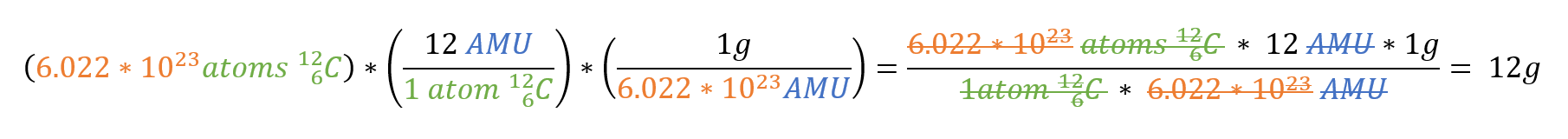Now that we know what a mole is and how it is used let’s work through some molarity questions that might come up while doing stoichiometry.

Here’s an example:

The lab manual says you need 3 moles of aluminum hydroxide [a white powder with the formula Al(OH)3] How many grams of aluminum hydroxide should you tell your lab partner to weigh out on the scale?

The first step is to find the mass of a molecule of aluminum hydroxide in AMU. The subscript at the bottom of the parentheses in the formula means that a single molecule contains 3 hydroxyl (OH) groups, making the total number of atoms in a single molecule 1 aluminum, 3 hydrogens, and 3 oxygens. On most periodic tables the average AMU for that element is listed below that elements name and symbol.  Looking at a periodic table we find the AMU of H is about 1, and the AMU of O is about 16, and Al is about 27. From here we can calculate the total mass of the molecule: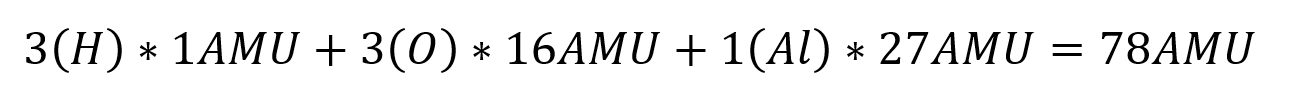Since the mass of an aluminum hydroxide molecule is 78 AMU/molecule, then the molar mass is 78 g/mole. However, the experiment is asking for 3 moles, so you should ask your lab partner for 234 g of aluminum hydroxide: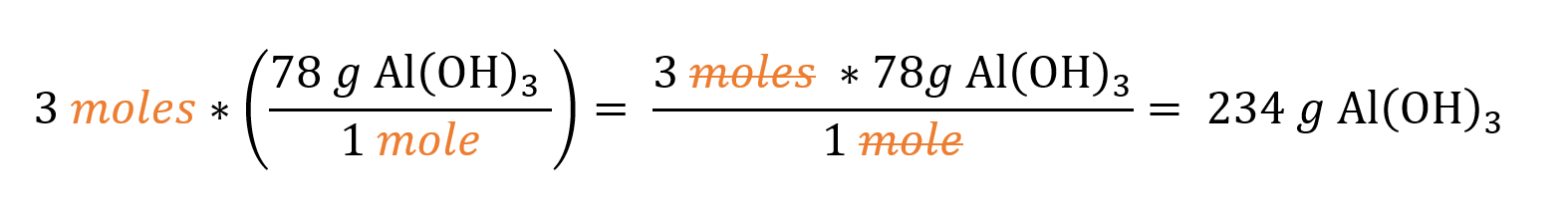Let’s look at one more:

After combusting a hydrocarbon in oxygen, the reaction leaves behind 90g of water.  How many moles of water did this reaction yield?

Like before, the first step is to calculate the AMU of a single molecule. The empirical formula for water is H2O meaning 2 Hydrogens, and one Oxygen. Returning to the periodic table we find the weight of Hydrogen is equal to about 1 AMU, and the weight of Oxygen is about 16 AMU so the total mass of one water molecule is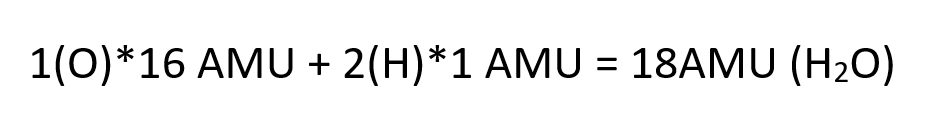If one water molecule has a mass of 18 AMU, then one mole of water molecules has a mass of 18g. That fact can be represented as a conversion factor: (1 mole H2O/18 g), now the only remaining step is to multiply the given mass of water at the start of the question by the conversion factor we just derived.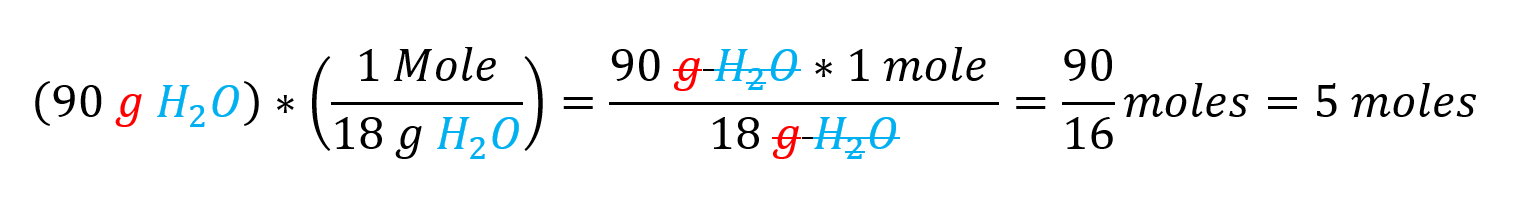The correct answer is that there are 5 moles of H2O molecules in 90 g of water.